# RS Aggarwal Solutions for Class 8 Maths Chapter 20 - Volume and Surface Area of Solids

RS Aggarwal Solutions For Class 8 Maths Chapter 20 Volume and Surface Area of Solids are provided here. You can download the pdf of RS Aggarwal Solutions for Class 8 Maths Chapter 20 Volume and Surface Area of Solids from the given links. RS Aggarwal Solutions helps students to attain a good score in the examinations, while also providing extensive knowledge about the subject, as Class 8 is a critical stage in their academic career. Therefore, we at BYJU’S provide answers to all questions uniquely and briefly.

The solutions curated by the faculty at BYJU’S provides the students with foundation to higher studies. RS Aggarwal Solutions for Class 8 Maths Chapter 20 Volume and Surface Area of Solids provides the concepts in an effective way.

## Download pdf of RS Aggarwal Solutions For Class 8 Maths Chapter 20 Volume and Surface Area of Solids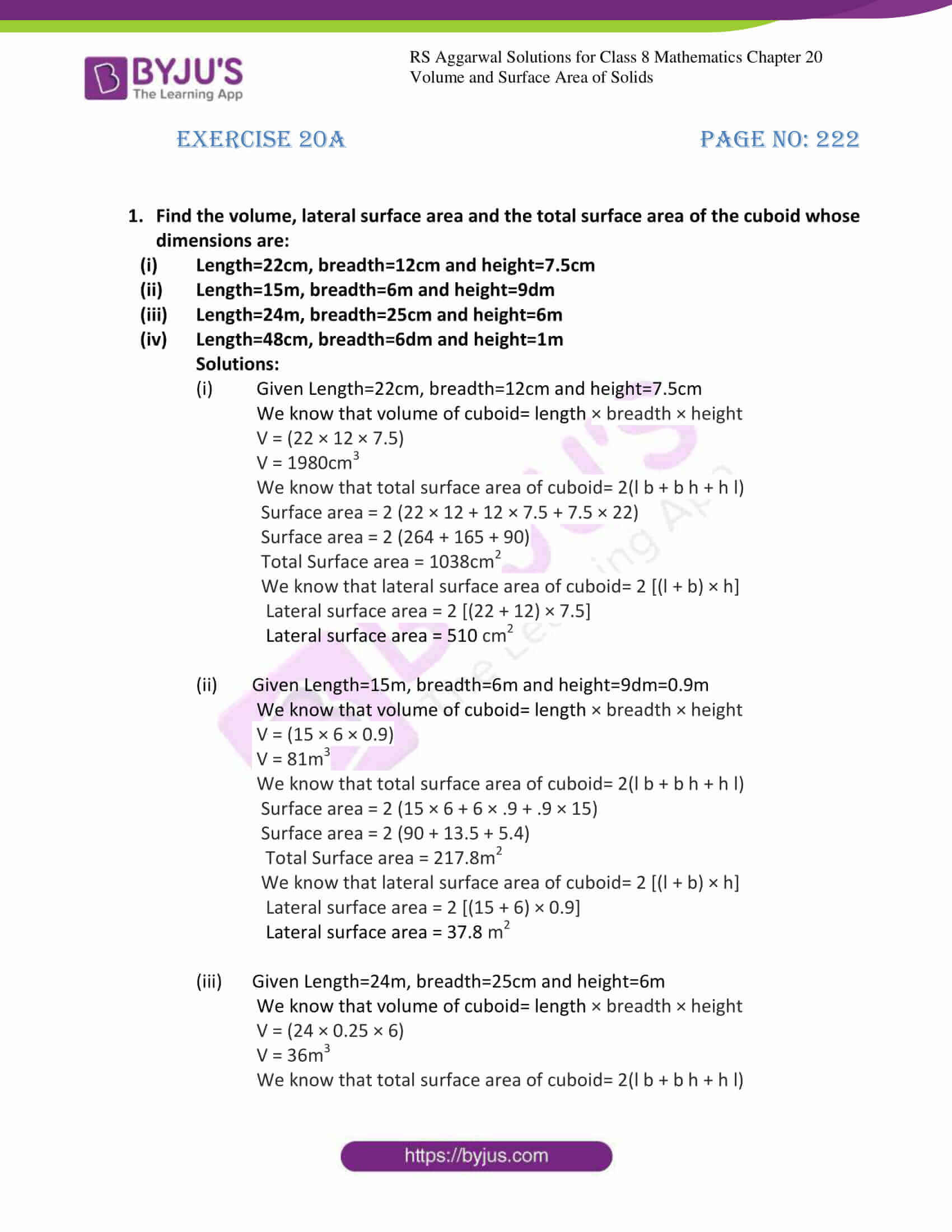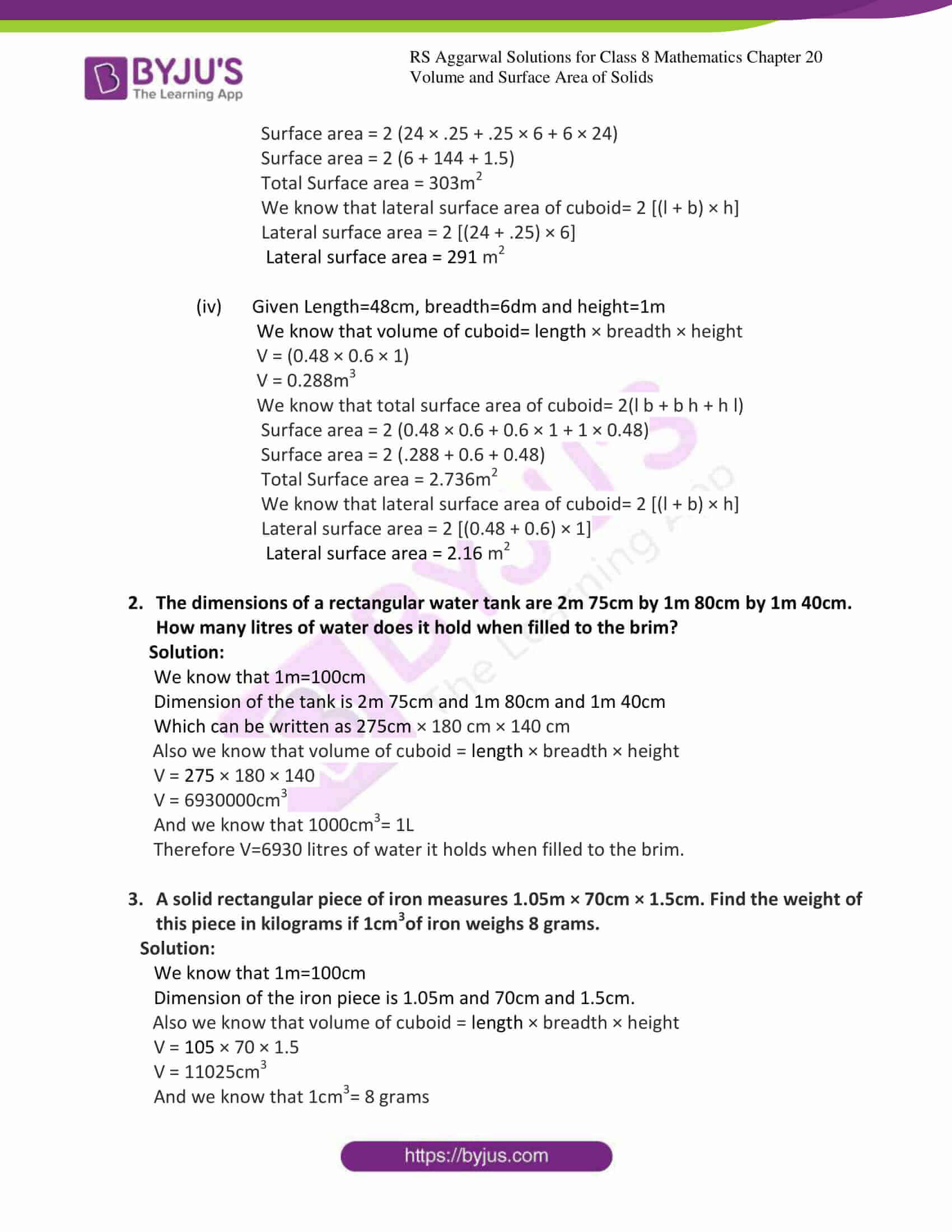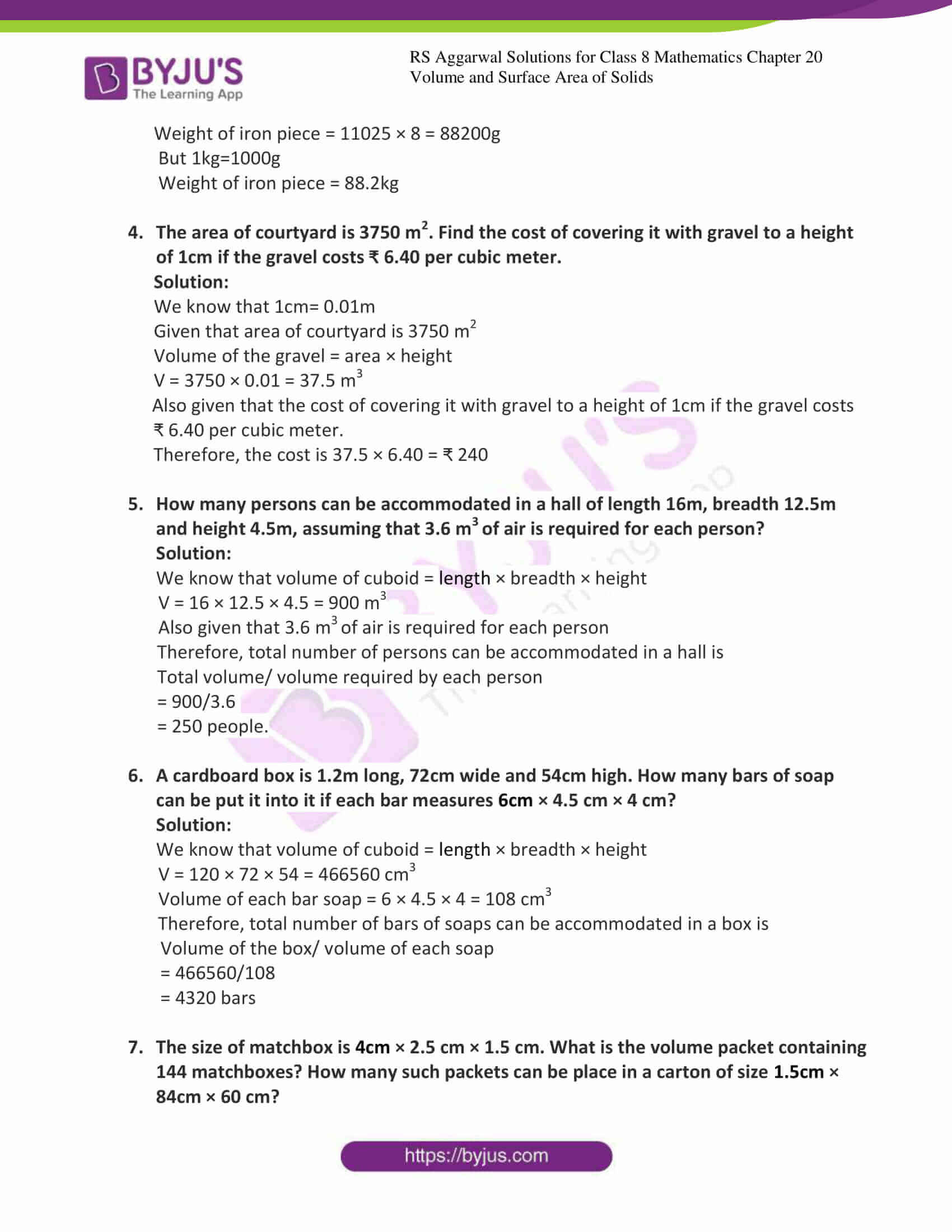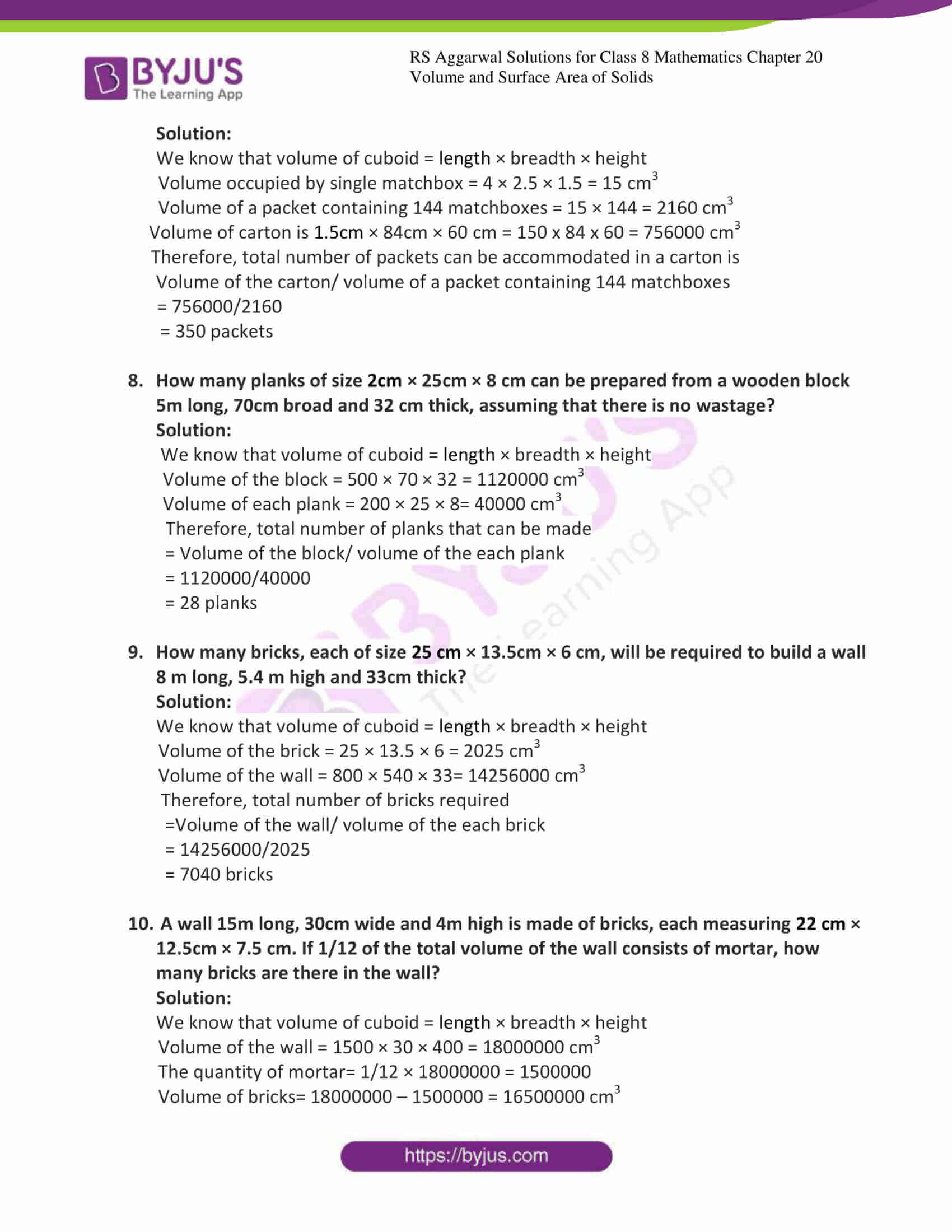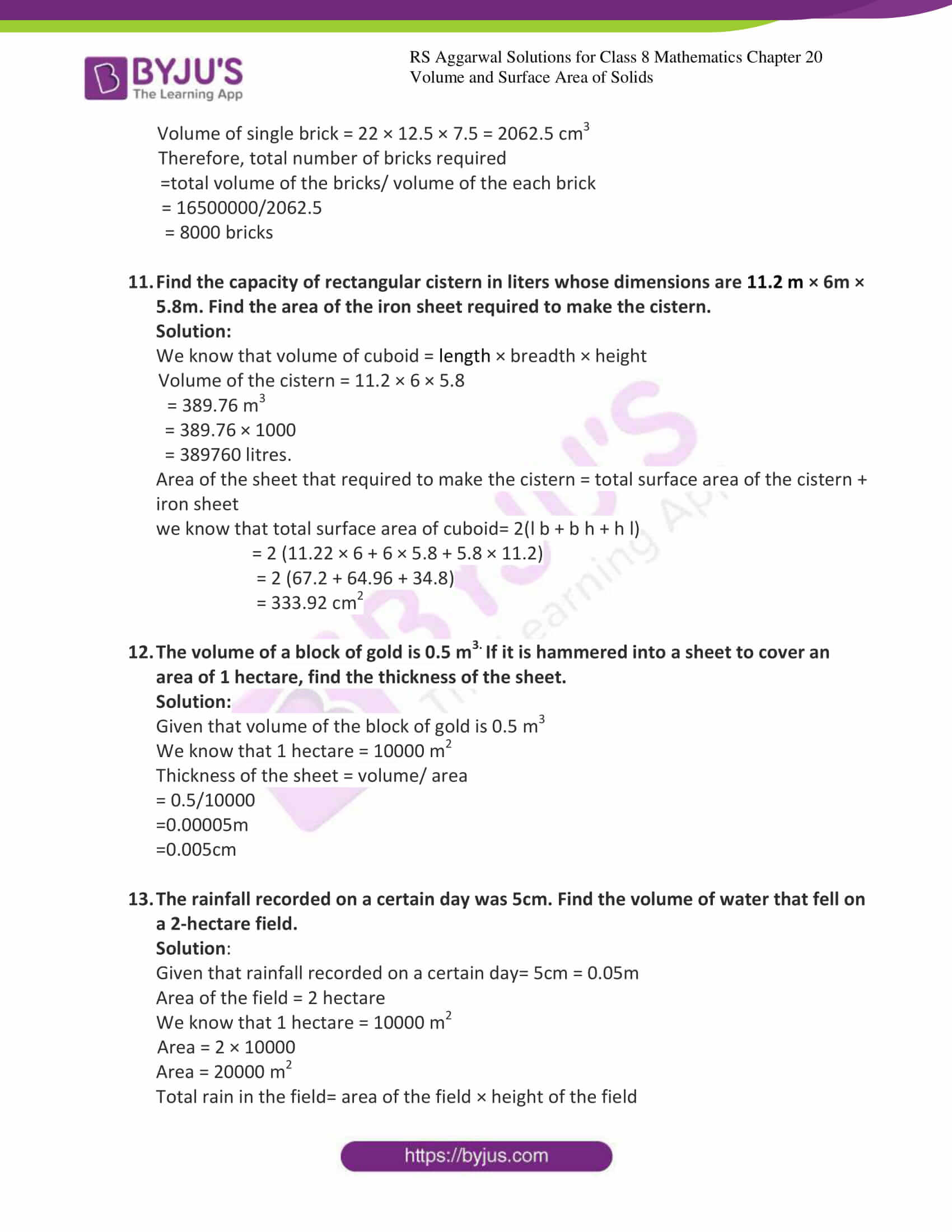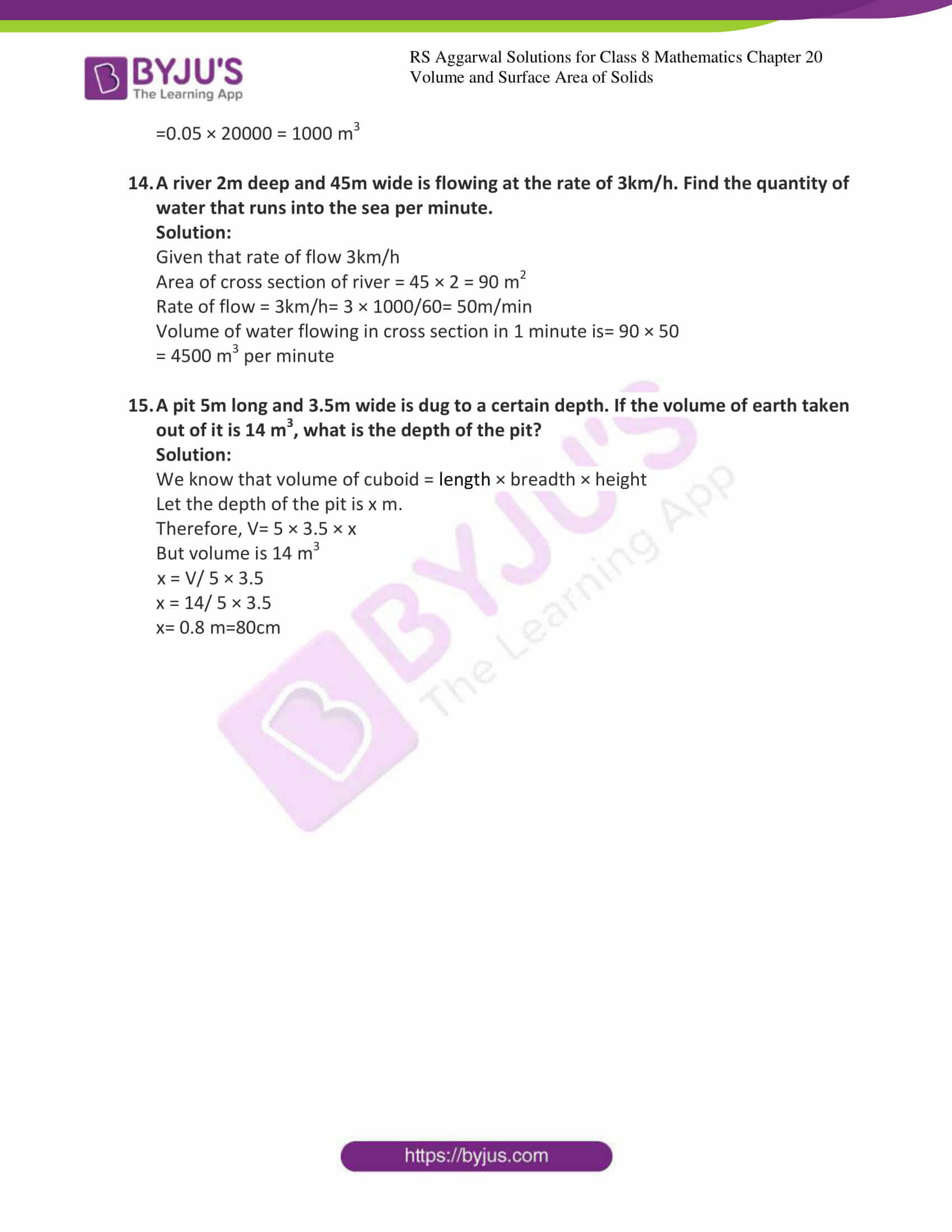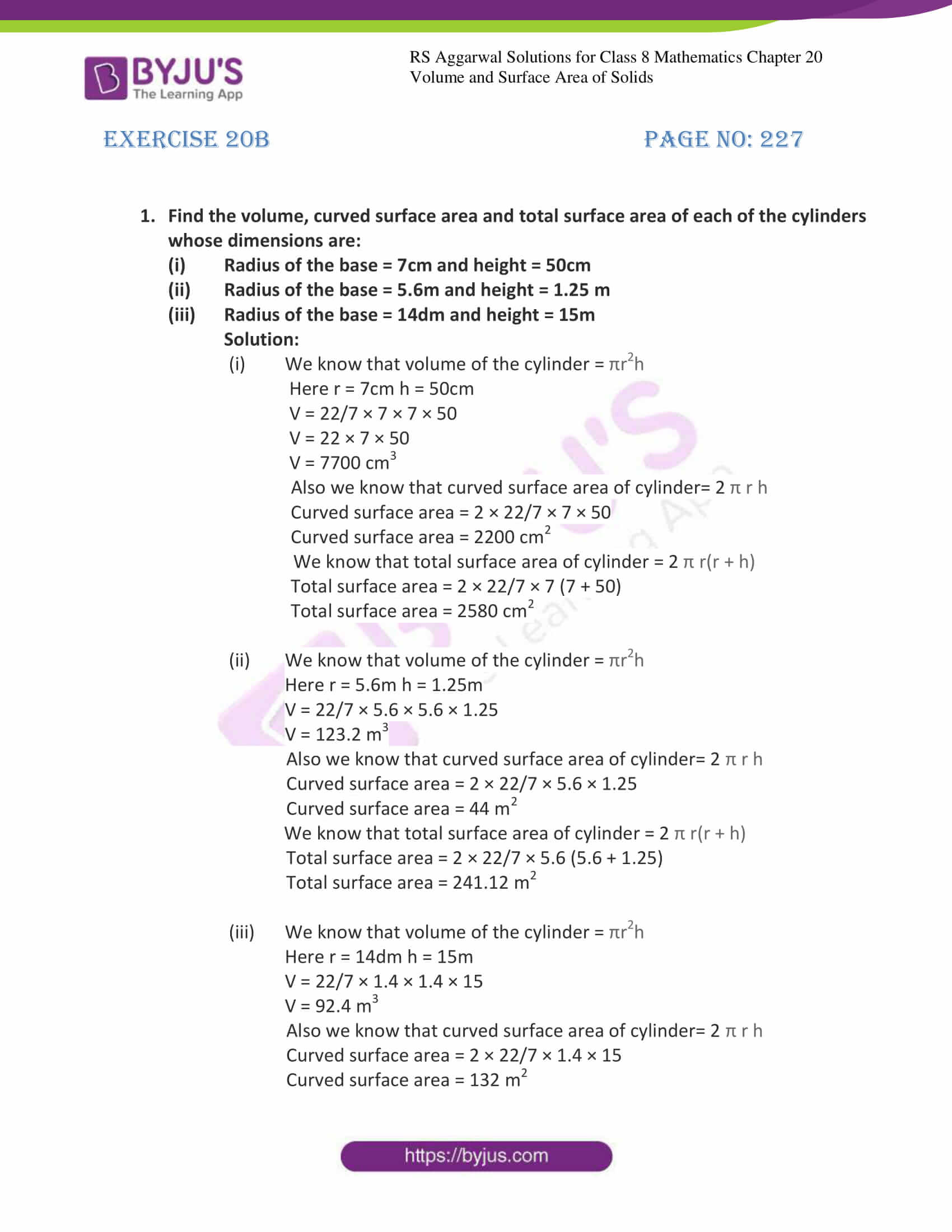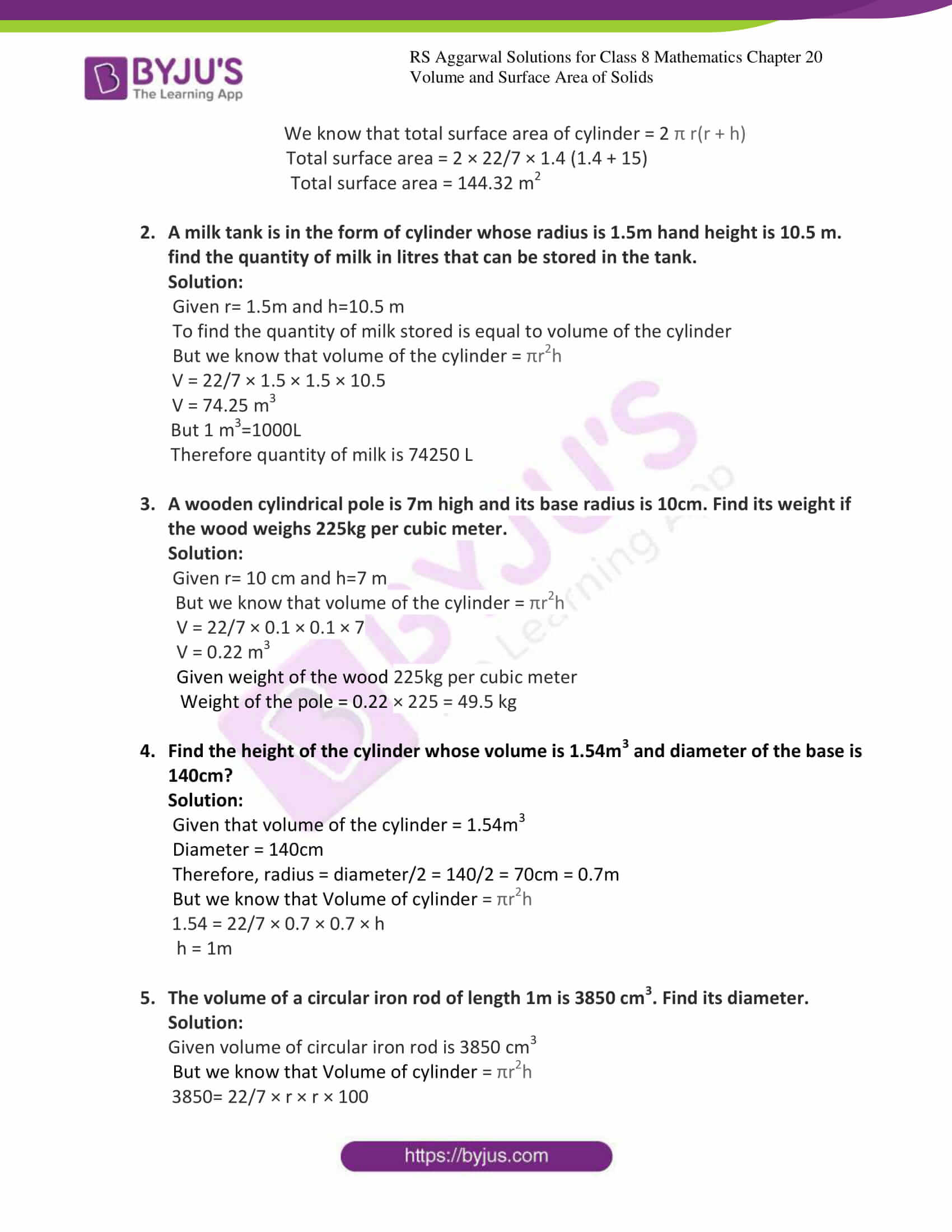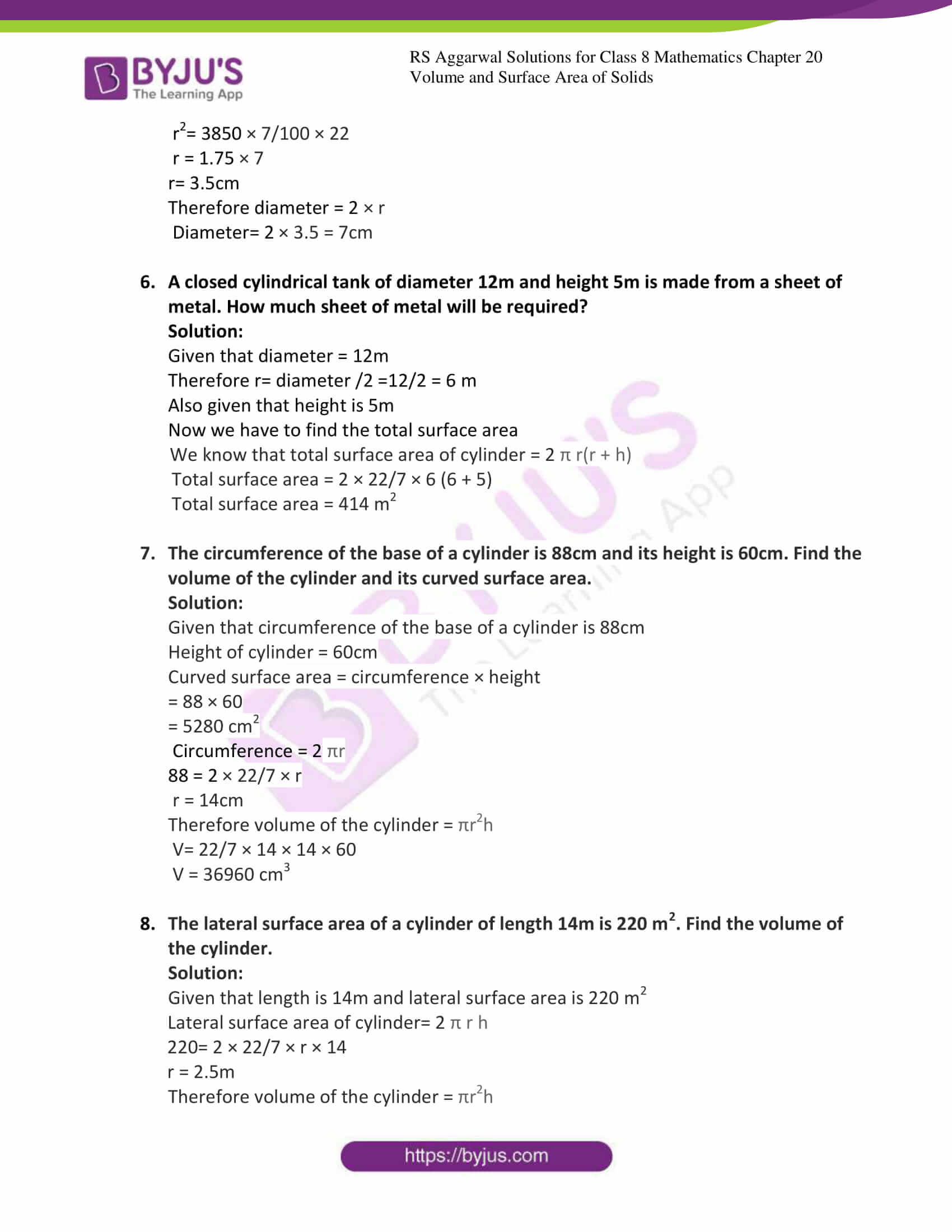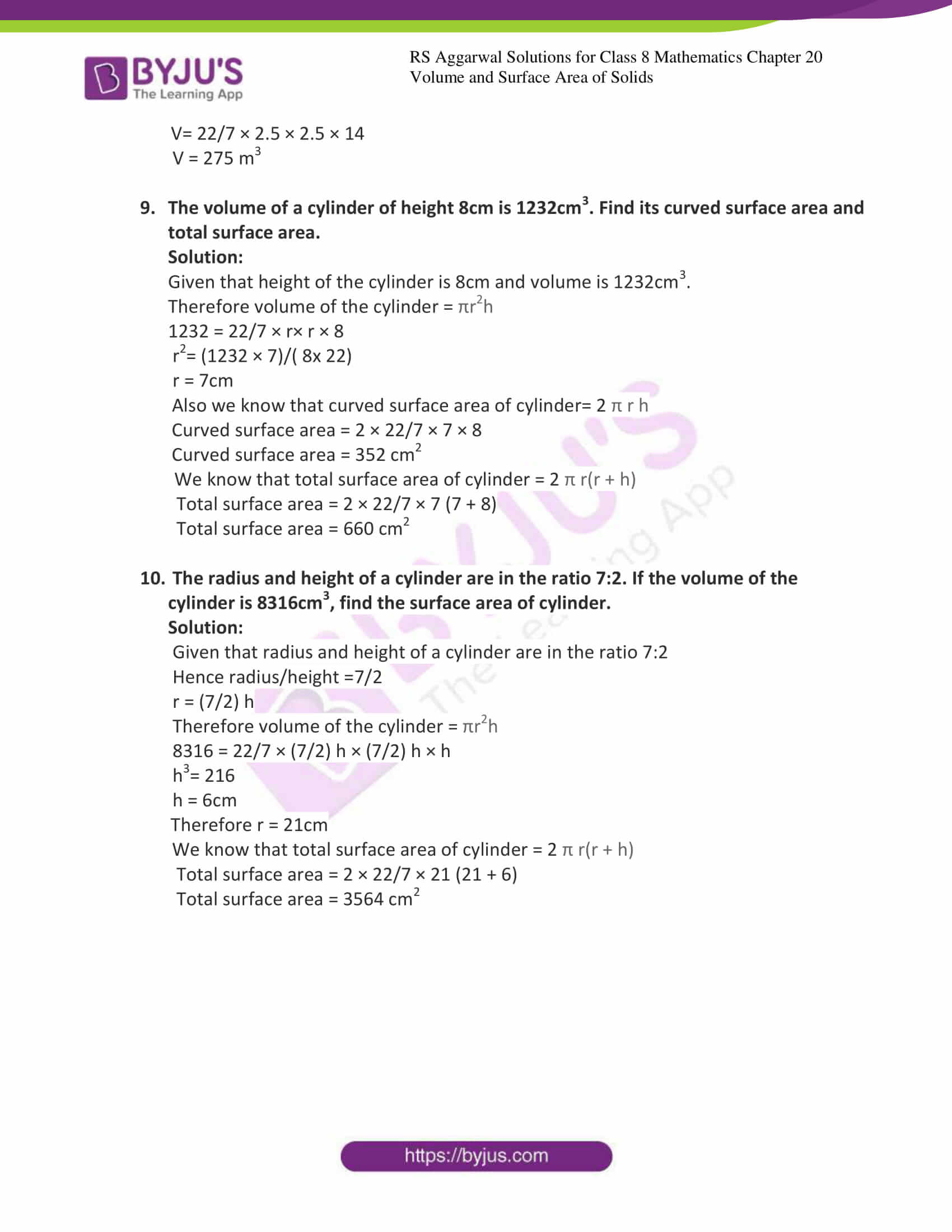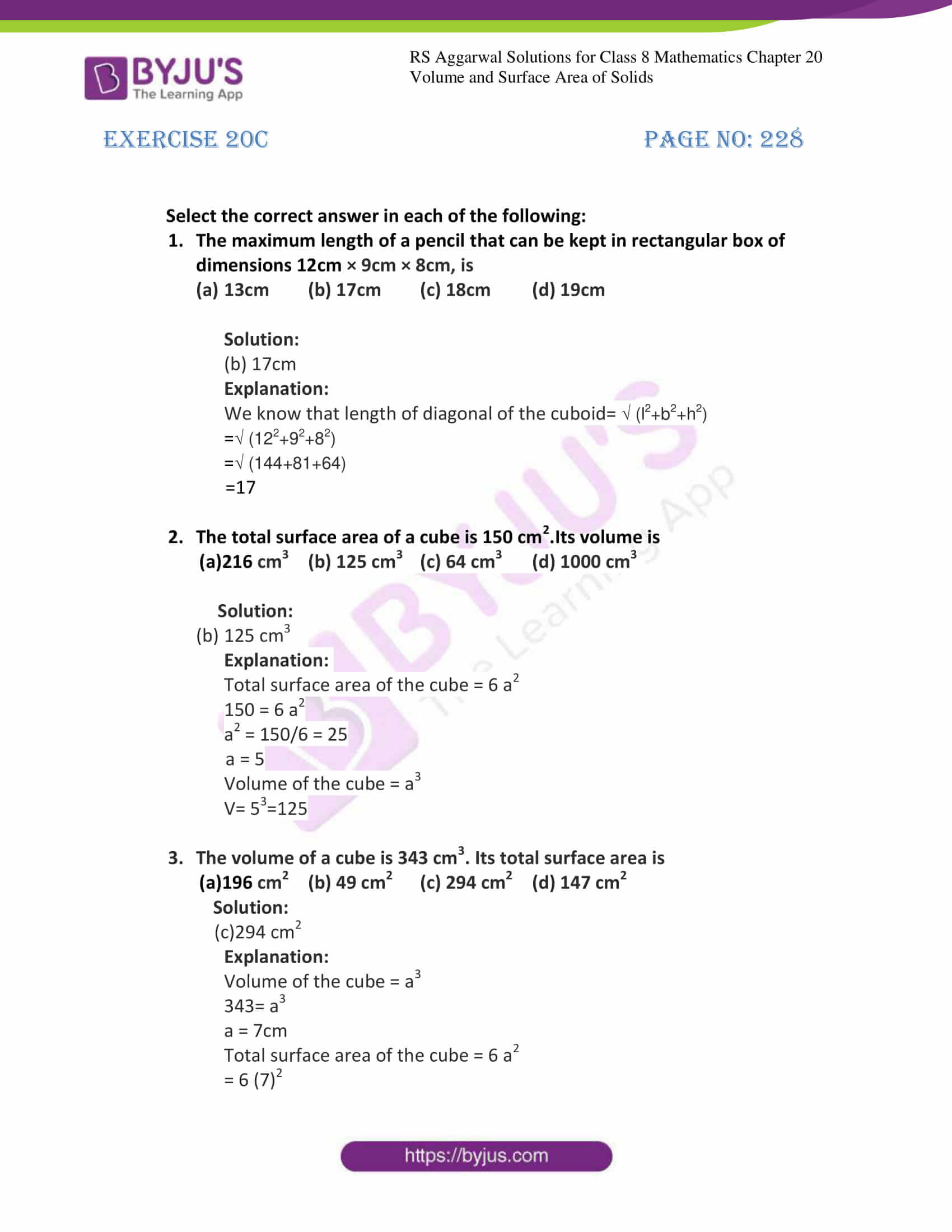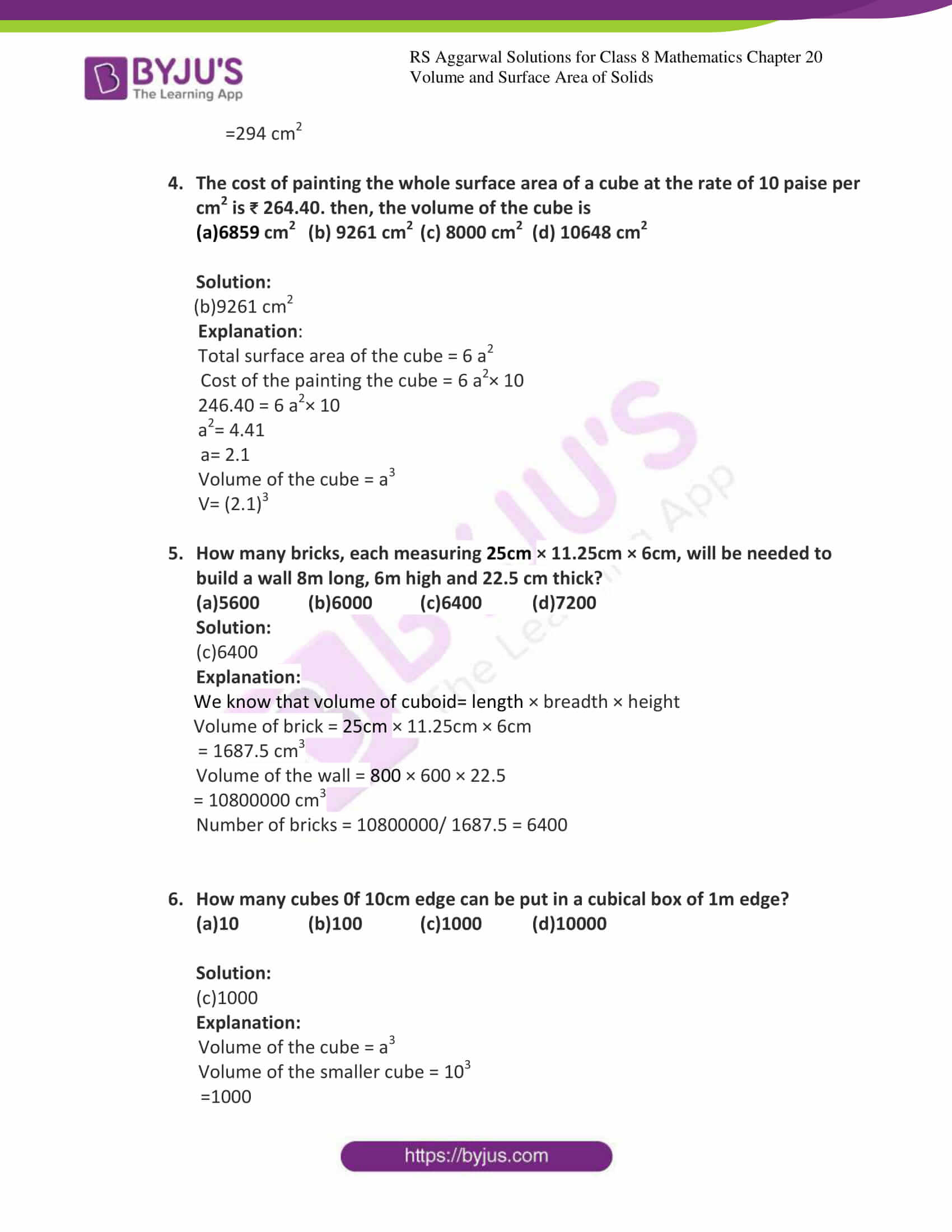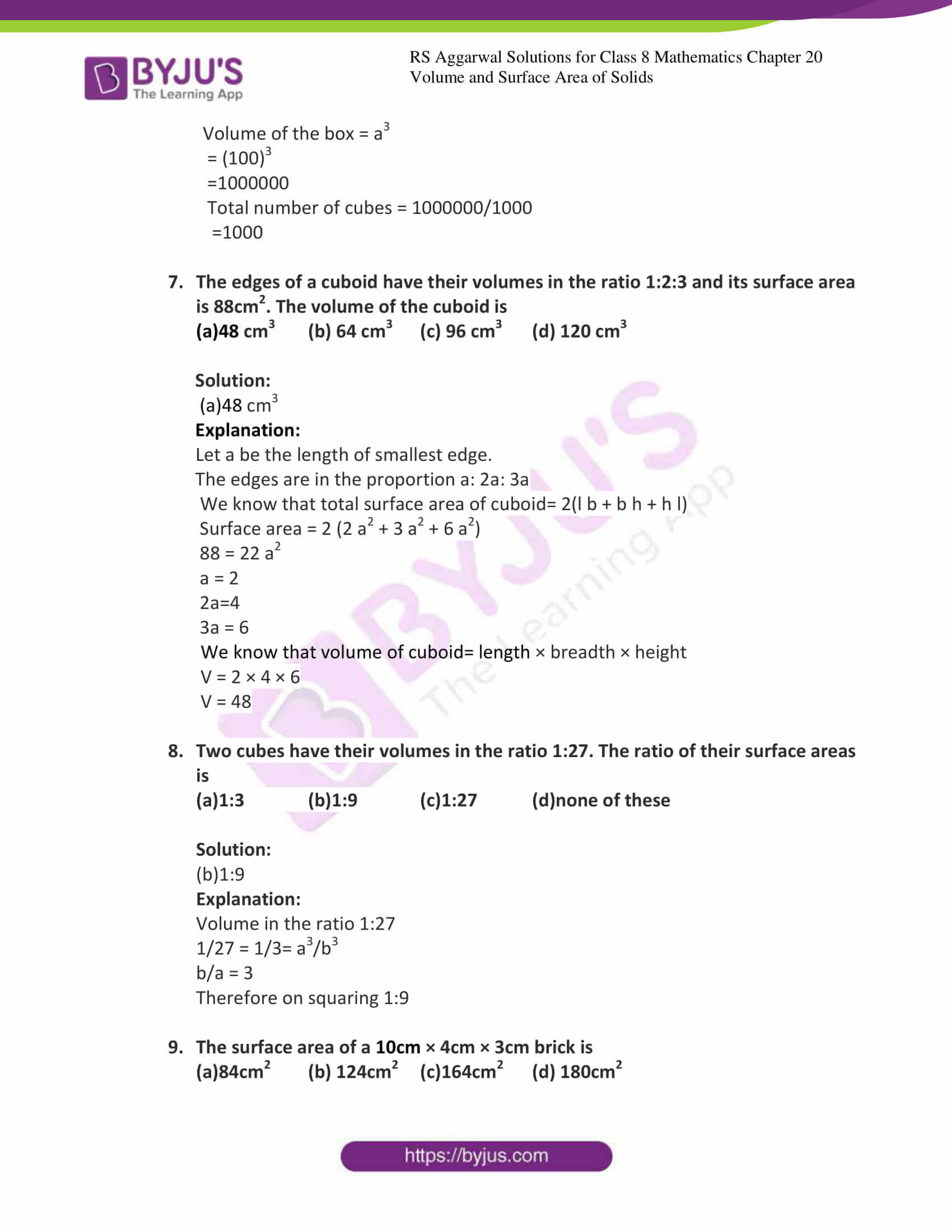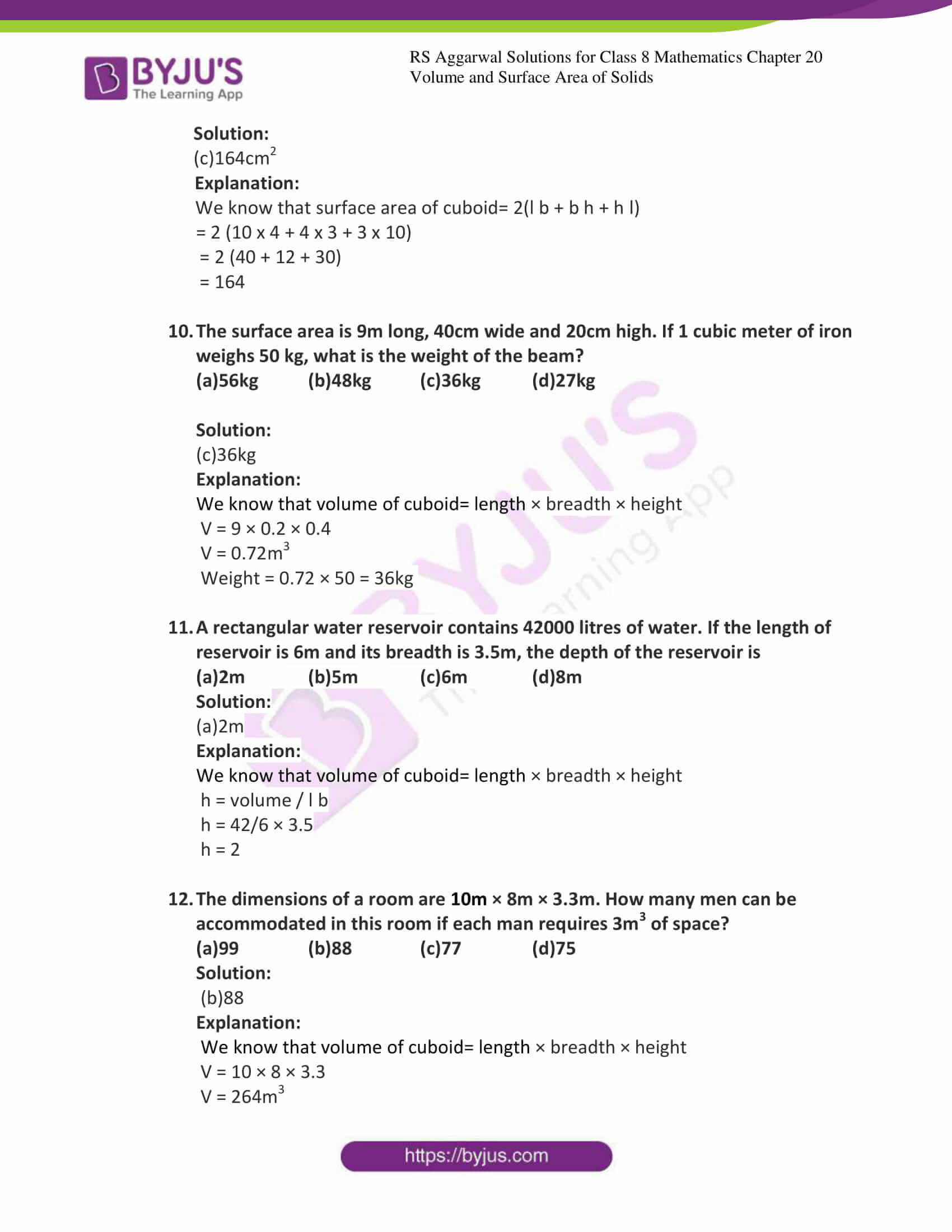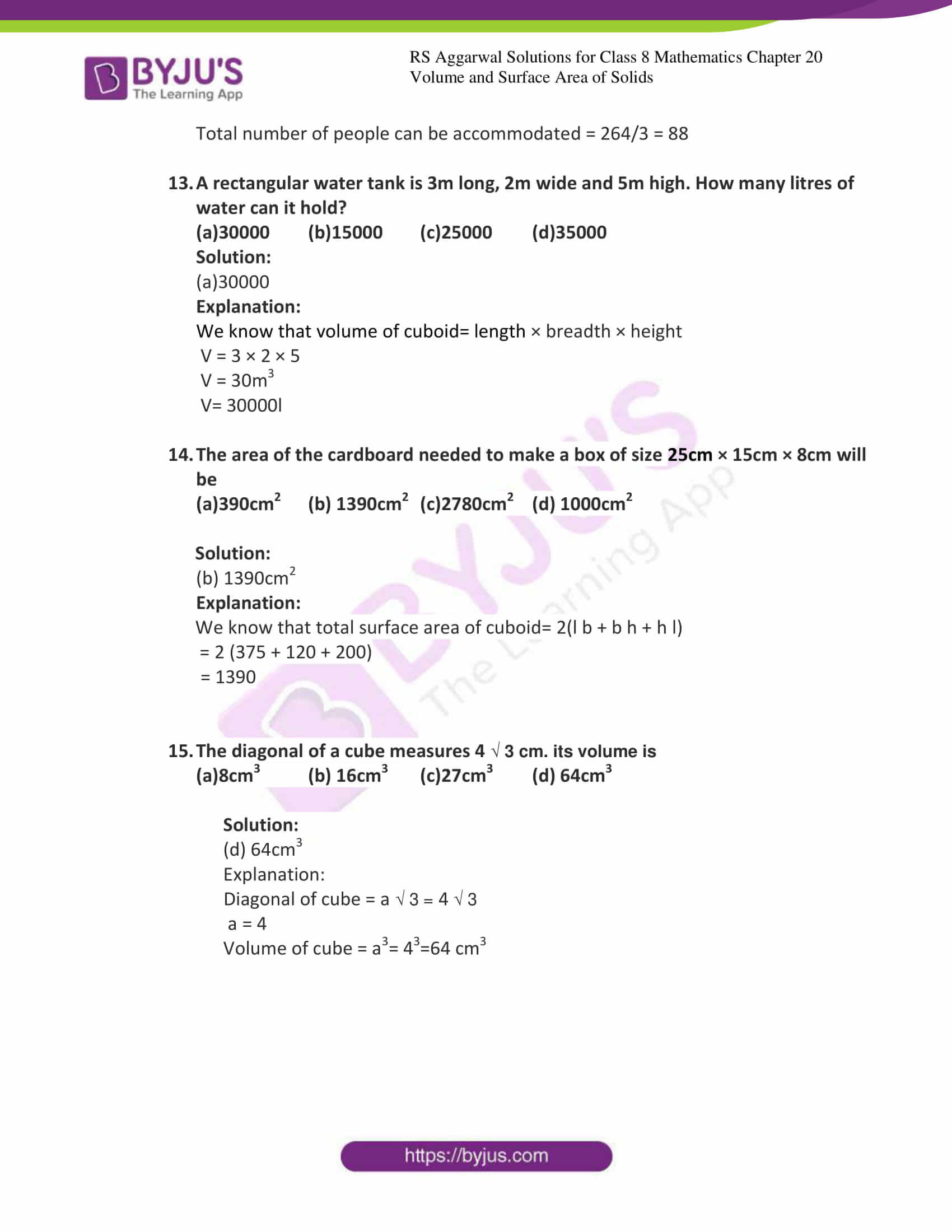### Access Answers to Maths RS Aggarwal Solutions for Class 8 Chapter 20 – Volume and Surface Area of Solids

Exercise 20A Page No: 222

1. Find the volume, lateral surface area and the total surface area of the cuboid whose dimensions are:

Solutions:

(i) Given Length=22cm, breadth=12cm and height=7.5cm

We know that volume of cuboid= length × breadth × height

V = (22 × 12 × 7.5)

V = 1980cm3

We know that total surface area of cuboid= 2(l b + b h + h l)

Surface area = 2 (22 × 12 + 12 × 7.5 + 7.5 × 22)

Surface area = 2 (264 + 165 + 90)

Total Surface area = 1038cm2

We know that lateral surface area of cuboid= 2 [(l + b) × h]

Lateral surface area = 2 [(22 + 12) × 7.5]

Lateral surface area = 510 cm2

(ii) Given Length=15m, breadth=6m and height=9dm=0.9m

We know that volume of cuboid= length × breadth × height

V = (15 × 6 × 0.9)

V = 81m3

We know that total surface area of cuboid= 2(l b + b h + h l)

Surface area = 2 (15 × 6 + 6 × .9 + .9 × 15)

Surface area = 2 (90 + 13.5 + 5.4)

Total Surface area = 217.8m2

We know that lateral surface area of cuboid= 2 [(l + b) × h]

Lateral surface area = 2 [(15 + 6) × 0.9]

Lateral surface area = 37.8 m2

(iii) Given Length=24m, breadth=25cm and height=6m

We know that volume of cuboid= length × breadth × height

V = (24 × 0.25 × 6)

V = 36m3

We know that total surface area of cuboid= 2(l b + b h + h l)

Surface area = 2 (24 × .25 + .25 × 6 + 6 × 24)

Surface area = 2 (6 + 144 + 1.5)

Total Surface area = 303m2

We know that lateral surface area of cuboid= 2 [(l + b) × h]

Lateral surface area = 2 [(24 + .25) × 6]

Lateral surface area = 291 m2

(iv) Given Length=48cm, breadth=6dm and height=1m

We know that volume of cuboid= length × breadth × height

V = (0.48 × 0.6 × 1)

V = 0.288m3

We know that total surface area of cuboid= 2(l b + b h + h l)

Surface area = 2 (0.48 × 0.6 + 0.6 × 1 + 1 × 0.48)

Surface area = 2 (.288 + 0.6 + 0.48)

Total Surface area = 2.736m2

We know that lateral surface area of cuboid= 2 [(l + b) × h]

Lateral surface area = 2 [(0.48 + 0.6) × 1]

Lateral surface area = 2.16 m2

2. The dimensions of a rectangular water tank are 2m 75cm by 1m 80cm by 1m 40cm. How many litres of water does it hold when filled to the brim?

Solution:

We know that 1m=100cm

Dimension of the tank is 2m 75cm and 1m 80cm and 1m 40cm

Which can be written as 275cm × 180 cm × 140 cm

Also we know that volume of cuboid = length × breadth × height

V = 275 × 180 × 140

V = 6930000cm3

And we know that 1000cm3= 1L

Therefore V=6930 litres of water it holds when filled to the brim.

3. A solid rectangular piece of iron measures 1.05m × 70cm × 1.5cm. Find the weight of this piece in kilograms if 1cm3of iron weighs 8 grams.

Solution:

We know that 1m=100cm

Dimension of the iron piece is 1.05m and 70cm and 1.5cm.

Also we know that volume of cuboid = length × breadth × height

V = 105 × 70 × 1.5

V = 11025cm3

And we know that 1cm3= 8 grams

Weight of iron piece = 11025 × 8 = 88200g

But 1kg=1000g

Weight of iron piece = 88.2kg

4. The area of courtyard is 3750 m2. Find the cost of covering it with gravel to a height of 1cm if the gravel costs ₹ 6.40 per cubic meter.

Solution:

We know that 1cm= 0.01m

Given that area of courtyard is 3750 m2

Volume of the gravel = area × height

V = 3750 × 0.01 = 37.5 m3

Also given that the cost of covering it with gravel to a height of 1cm if the gravel costs

₹ 6.40 per cubic meter.

Therefore, the cost is 37.5 × 6.40 = ₹ 240

5. How many persons can be accommodated in a hall of length 16m, breadth 12.5m and height 4.5m, assuming that 3.6 m3 of air is required for each person?

Solution:

We know that volume of cuboid = length × breadth × height

V = 16 × 12.5 × 4.5 = 900 m3

Also given that 3.6 m3 of air is required for each person

Therefore, total number of persons can be accommodated in a hall is

Total volume/ volume required by each person

= 900/3.6

= 250 people.

6. A cardboard box is 1.2m long, 72cm wide and 54cm high. How many bars of soap can be put it into it if each bar measures 6cm × 4.5 cm × 4 cm?

Solution:

We know that volume of cuboid = length × breadth × height

V = 120 × 72 × 54 = 466560 cm3

Volume of each bar soap = 6 × 4.5 × 4 = 108 cm3

Therefore, total number of bars of soaps can be accommodated in a box is

Volume of the box/ volume of each soap

= 466560/108

= 4320 bars

7. The size of matchbox is 4cm × 2.5 cm × 1.5 cm. What is the volume packet containing 144 matchboxes? How many such packets can be place in a carton of size 1.5cm × 84cm × 60 cm?

Solution:

We know that volume of cuboid = length × breadth × height

Volume occupied by single matchbox = 4 × 2.5 × 1.5 = 15 cm3

Volume of a packet containing 144 matchboxes = 15 × 144 = 2160 cm3

Volume of carton is 1.5cm × 84cm × 60 cm = 150 x 84 x 60 = 756000 cm3

Therefore, total number of packets can be accommodated in a carton is

Volume of the carton/ volume of a packet containing 144 matchboxes

= 756000/2160

= 350 packets

8. How many planks of size 2cm × 25cm × 8 cm can be prepared from a wooden block 5m long, 70cm broad and 32 cm thick, assuming that there is no wastage?

Solution:

We know that volume of cuboid = length × breadth × height

Volume of the block = 500 × 70 × 32 = 1120000 cm3

Volume of each plank = 200 × 25 × 8= 40000 cm3

Therefore, total number of planks that can be made

= Volume of the block/ volume of the each plank

= 1120000/40000

= 28 planks

9. How many bricks, each of size 25 cm × 13.5cm × 6 cm, will be required to build a wall 8 m long, 5.4 m high and 33cm thick?

Solution:

We know that volume of cuboid = length × breadth × height

Volume of the brick = 25 × 13.5 × 6 = 2025 cm3

Volume of the wall = 800 × 540 × 33= 14256000 cm3

Therefore, total number of bricks required

=Volume of the wall/ volume of the each brick

= 14256000/2025

= 7040 bricks

10. A wall 15m long, 30cm wide and 4m high is made of bricks, each measuring 22 cm × 12.5cm × 7.5 cm. If 1/12 of the total volume of the wall consists of mortar, how many bricks are there in the wall?

Solution:

We know that volume of cuboid = length × breadth × height

Volume of the wall = 1500 × 30 × 400 = 18000000 cm3

The quantity of mortar= 1/12 × 18000000 = 1500000

Volume of bricks= 18000000 – 1500000 = 16500000 cm3

Volume of single brick = 22 × 12.5 × 7.5 = 2062.5 cm3

Therefore, total number of bricks required

=total volume of the bricks/ volume of the each brick

= 16500000/2062.5

= 8000 bricks

11. Find the capacity of rectangular cistern in liters whose dimensions are 11.2 m × 6m × 5.8m. Find the area of the iron sheet required to make the cistern.

Solution:

We know that volume of cuboid = length × breadth × height

Volume of the cistern = 11.2 × 6 × 5.8

= 389.76 m3

= 389.76 × 1000

= 389760 litres.

Area of the sheet that required to make the cistern = total surface area of the cistern + iron sheet

we know that total surface area of cuboid= 2(l b + b h + h l)

= 2 (11.22 × 6 + 6 × 5.8 + 5.8 × 11.2)

= 2 (67.2 + 64.96 + 34.8)

= 333.92 cm2

12. The volume of a block of gold is 0.5 m3. If it is hammered into a sheet to cover an area of 1 hectare, find the thickness of the sheet.

Solution:

Given that volume of the block of gold is 0.5 m3

We know that 1 hectare = 10000 m2

Thickness of the sheet = volume/ area

= 0.5/10000

=0.00005m

=0.005cm

13. The rainfall recorded on a certain day was 5cm. Find the volume of water that fell on a 2-hectare field.

Solution:

Given that rainfall recorded on a certain day= 5cm = 0.05m

Area of the field = 2 hectare

We know that 1 hectare = 10000 m2

Area = 2 × 10000

Area = 20000 m2

Total rain in the field= area of the field × height of the field

=0.05 × 20000 = 1000 m3

14. A river 2m deep and 45m wide is flowing at the rate of 3km/h. Find the quantity of water that runs into the sea per minute.

Solution:

Given that rate of flow 3km/h

Area of cross section of river = 45 × 2 = 90 m2

Rate of flow = 3km/h= 3 × 1000/60= 50m/min

Volume of water flowing in cross section in 1 minute is= 90 × 50

= 4500 m3 per minute

15. A pit 5m long and 3.5m wide is dug to a certain depth. If the volume of earth taken out of it is 14 m3, what is the depth of the pit?

Solution:

We know that volume of cuboid = length × breadth × height

Let the depth of the pit is x m.

Therefore, V= 5 × 3.5 × x

But volume is 14 m3

x = V/ 5 × 3.5

x = 14/ 5 × 3.5

x= 0.8 m=80cm

Exercise 20B Page No: 227

1. Find the volume, curved surface area and total surface area of each of the cylinders whose dimensions are:

(i) Radius of the base = 7cm and height = 50cm

(ii) Radius of the base = 5.6m and height = 1.25 m

(iii) Radius of the base = 14dm and height = 15m

Solution:

(i) We know that volume of the cylinder = πr2h

Here r = 7cm h = 50cm

V = 22/7 × 7 × 7 × 50

V = 22 × 7 × 50

V = 7700 cm3

Also we know that curved surface area of cylinder= 2 π r h

Curved surface area = 2 × 22/7 × 7 × 50

Curved surface area = 2200 cm2

We know that total surface area of cylinder = 2 π r(r + h)

Total surface area = 2 × 22/7 × 7 (7 + 50)

Total surface area = 2580 cm2

(ii) We know that volume of the cylinder = πr2h

Here r = 5.6m h = 1.25m

V = 22/7 × 5.6 × 5.6 × 1.25

V = 123.2 m3

Also we know that curved surface area of cylinder= 2 π r h

Curved surface area = 2 × 22/7 × 5.6 × 1.25

Curved surface area = 44 m2

We know that total surface area of cylinder = 2 π r(r + h)

Total surface area = 2 × 22/7 × 5.6 (5.6 + 1.25)

Total surface area = 241.12 m2

(iii) We know that volume of the cylinder = πr2h

Here r = 14dm h = 15m

V = 22/7 × 1.4 × 1.4 × 15

V = 92.4 m3

Also we know that curved surface area of cylinder= 2 π r h

Curved surface area = 2 × 22/7 × 1.4 × 15

Curved surface area = 132 m2

We know that total surface area of cylinder = 2 π r(r + h)

Total surface area = 2 × 22/7 × 1.4 (1.4 + 15)

Total surface area = 144.32 m2

2. A milk tank is in the form of cylinder whose radius is 1.5m hand height is 10.5 m. find the quantity of milk in litres that can be stored in the tank.

Solution:

Given r= 1.5m and h=10.5 m

To find the quantity of milk stored is equal to volume of the cylinder

But we know that volume of the cylinder = πr2h

V = 22/7 × 1.5 × 1.5 × 10.5

V = 74.25 m3

But 1 m3=1000L

Therefore quantity of milk is 74250 L

3. A wooden cylindrical pole is 7m high and its base radius is 10cm. Find its weight if the wood weighs 225kg per cubic meter.

Solution:

Given r= 10 cm and h=7 m

But we know that volume of the cylinder = πr2h

V = 22/7 × 0.1 × 0.1 × 7

V = 0.22 m3

Given weight of the wood 225kg per cubic meter

Weight of the pole = 0.22 × 225 = 49.5 kg

4. Find the height of the cylinder whose volume is 1.54m3 and diameter of the base is 140cm?

Solution:

Given that volume of the cylinder = 1.54m3

Diameter = 140cm

Therefore, radius = diameter/2 = 140/2 = 70cm = 0.7m

But we know that Volume of cylinder = πr2h

1.54 = 22/7 × 0.7 × 0.7 × h

h = 1m

5. The volume of a circular iron rod of length 1m is 3850 cm3. Find its diameter.

Solution:

Given volume of circular iron rod is 3850 cm3

But we know that Volume of cylinder = πr2h

3850= 22/7 × r × r × 100

r2= 3850 × 7/100 × 22

r = 1.75 × 7

r= 3.5cm

Therefore diameter = 2 × r

Diameter= 2 × 3.5 = 7cm

6. A closed cylindrical tank of diameter 12m and height 5m is made from a sheet of metal. How much sheet of metal will be required?

Solution:

Given that diameter = 12m

Therefore r= diameter /2 =12/2 = 6 m

Also given that height is 5m

Now we have to find the total surface area

We know that total surface area of cylinder = 2 π r(r + h)

Total surface area = 2 × 22/7 × 6 (6 + 5)

Total surface area = 414 m2

7. The circumference of the base of a cylinder is 88cm and its height is 60cm. Find the volume of the cylinder and its curved surface area.

Solution:

Given that circumference of the base of a cylinder is 88cm

Height of cylinder = 60cm

Curved surface area = circumference × height

= 88 × 60

= 5280 cm2

Circumference = 2 πr

88 = 2 × 22/7 × r

r = 14cm

Therefore volume of the cylinder = πr2h

V= 22/7 × 14 × 14 × 60

V = 36960 cm3

8. The lateral surface area of a cylinder of length 14m is 220 m2. Find the volume of the cylinder.

Solution:

Given that length is 14m and lateral surface area is 220 m2

Lateral surface area of cylinder= 2 π r h

220= 2 × 22/7 × r × 14

r = 2.5m

Therefore volume of the cylinder = πr2h

V= 22/7 × 2.5 × 2.5 × 14

V = 275 m3

9. The volume of a cylinder of height 8cm is 1232cm3. Find its curved surface area and total surface area.

Solution:

Given that height of the cylinder is 8cm and volume is 1232cm3.

Therefore volume of the cylinder = πr2h

1232 = 22/7 × r× r × 8

r2= (1232 × 7)/( 8x 22)

r = 7cm

Also we know that curved surface area of cylinder= 2 π r h

Curved surface area = 2 × 22/7 × 7 × 8

Curved surface area = 352 cm2

We know that total surface area of cylinder = 2 π r(r + h)

Total surface area = 2 × 22/7 × 7 (7 + 8)

Total surface area = 660 cm2

10. The radius and height of a cylinder are in the ratio 7:2. If the volume of the cylinder is 8316cm3, find the surface area of cylinder.

Solution:

Given that radius and height of a cylinder are in the ratio 7:2

r = (7/2) h

Therefore volume of the cylinder = πr2h

8316 = 22/7 × (7/2) h × (7/2) h × h

h3= 216

h = 6cm

Therefore r = 21cm

We know that total surface area of cylinder = 2 π r(r + h)

Total surface area = 2 × 22/7 × 21 (21 + 6)

Total surface area = 3564 cm2

Exercise 20C Page No: 228

Select the correct answer in each of the following:

1. The maximum length of a pencil that can be kept in rectangular box of dimensions 12cm × 9cm × 8cm, is

(a) 13cm (b) 17cm (c) 18cm (d) 19cm

Solution:

(b) 17cm

Explanation:

We know that length of diagonal of the cuboid= √ (l2+b2+h2)

=√ (122+92+82)

=√ (144+81+64)

=17

2. The total surface area of a cube is 150 cm2.Its volume is

(a)216 cm3 (b) 125 cm3 (c) 64 cm3 (d) 1000 cm3

Solution:

1. 125 cm3

Explanation:

Total surface area of the cube = 6 a2

150 = 6 a2

a2 = 150/6 = 25

a = 5

Volume of the cube = a3

V= 53=125

3. The volume of a cube is 343 cm3. Its total surface area is

(a)196 cm2 (b) 49 cm2 (c) 294 cm2 (d) 147 cm2

Solution:

Explanation:

Volume of the cube = a3

343= a3

a = 7cm

Total surface area of the cube = 6 a2

= 6 (7)2

=294 cm2

4. The cost of painting the whole surface area of a cube at the rate of 10 paise per cm2 is ₹ 264.40. then, the volume of the cube is

(a)6859 cm2 (b) 9261 cm2 (c) 8000 cm2 (d) 10648 cm2

Solution:

(b)9261 cm2

Explanation:

Total surface area of the cube = 6 a2

Cost of the painting the cube = 6 a2× 10

246.40 = 6 a2× 10

a2= 4.41

a= 2.1

Volume of the cube = a3

V= (2.1)3

5. How many bricks, each measuring 25cm × 11.25cm × 6cm, will be needed to build a wall 8m long, 6m high and 22.5 cm thick?

(a)5600 (b)6000 (c)6400 (d)7200

Solution:

Explanation:

We know that volume of cuboid= length × breadth × height

Volume of brick = 25cm × 11.25cm × 6cm

= 1687.5 cm3

Volume of the wall = 800 × 600 × 22.5

= 10800000 cm3

Number of bricks = 10800000/ 1687.5 = 6400

6. How many cubes 0f 10cm edge can be put in a cubical box of 1m edge?

(a)10 (b)100 (c)1000 (d)10000

Solution:

Explanation:

Volume of the cube = a3

Volume of the smaller cube = 103

=1000

Volume of the box = a3

= (100)3

=1000000

Total number of cubes = 1000000/1000

=1000

7. The edges of a cuboid have their volumes in the ratio 1:2:3 and its surface area is 88cm2. The volume of the cuboid is

(a)48 cm3 (b) 64 cm3 (c) 96 cm3 (d) 120 cm3

Solution:

(a)48 cm3

Explanation:

Let a be the length of smallest edge.

The edges are in the proportion a: 2a: 3a

We know that total surface area of cuboid= 2(l b + b h + h l)

Surface area = 2 (2 a2 + 3 a2 + 6 a2)

88 = 22 a2

a = 2

2a=4

3a = 6

We know that volume of cuboid= length × breadth × height

V = 2 × 4 × 6

V = 48

8. Two cubes have their volumes in the ratio 1:27. The ratio of their surface areas is

(a)1:3 (b)1:9 (c)1:27 (d)none of these

Solution:

(b)1:9

Explanation:

Volume in the ratio 1:27

1/27 = 1/3= a3/b3

b/a = 3

Therefore on squaring 1:9

9. The surface area of a 10cm × 4cm × 3cm brick is

(a)84cm2 (b) 124cm2 (c)164cm2 (d) 180cm2

Solution:

Explanation:

We know that surface area of cuboid= 2(l b + b h + h l)

= 2 (10 x 4 + 4 x 3 + 3 x 10)

= 2 (40 + 12 + 30)

= 164

10. The surface area is 9m long, 40cm wide and 20cm high. If 1 cubic meter of iron weighs 50 kg, what is the weight of the beam?

(a)56kg (b)48kg (c)36kg (d)27kg

Solution:

Explanation:

We know that volume of cuboid= length × breadth × height

V = 9 × 0.2 × 0.4

V = 0.72m3

Weight = 0.72 × 50 = 36kg

11. A rectangular water reservoir contains 42000 litres of water. If the length of reservoir is 6m and its breadth is 3.5m, the depth of the reservoir is

(a)2m (b)5m (c)6m (d)8m

Solution:

(a)2m

Explanation:

We know that volume of cuboid= length × breadth × height

h = volume / l b

h = 42/6 × 3.5

h = 2

12. The dimensions of a room are 10m × 8m × 3.3m. How many men can be accommodated in this room if each man requires 3m3 of space?

(a)99 (b)88 (c)77 (d)75

Solution:

(b)88

Explanation:

We know that volume of cuboid= length × breadth × height

V = 10 × 8 × 3.3

V = 264m3

Total number of people can be accommodated = 264/3 = 88

13. A rectangular water tank is 3m long, 2m wide and 5m high. How many litres of water can it hold?

(a)30000 (b)15000 (c)25000 (d)35000

Solution:

(a)30000

Explanation:

We know that volume of cuboid= length × breadth × height

V = 3 × 2 × 5

V = 30m3

V= 30000l

14. The area of the cardboard needed to make a box of size 25cm × 15cm × 8cm will be

(a)390cm2 (b) 1390cm2 (c)2780cm2 (d) 1000cm2

Solution:

1. 1390cm2

Explanation:

We know that total surface area of cuboid= 2(l b + b h + h l)

= 2 (375 + 120 + 200)

= 1390

15. The diagonal of a cube measures 4 √ 3 cm. its volume is

(a)8cm3 (b) 16cm3 (c)27cm3 (d) 64cm3

Solution:

(d) 64cm3

Explanation:

Diagonal of cube = a √ 3 = 4 √ 3

a = 4

Volume of cube = a3= 43=64 cm3

## RS Aggarwal Solutions for Class 8 Maths Chapter 20- Volume and Surface Area of Solids

Chapter 20, Volume and Surface Area of Solids , contains 3 Exercises. RS Aggarwal Solutions given here contains the answers to all the questions present in these exercises. Let us have a look at some of the concepts that are being discussed in this Chapter.

Definition of Solids

Definition of cube and its properties

Volume of a solid

Definition of cuboid and its properties

Standard unit of Volume

Formula for Volume of a cube

Formula for Surface Area of a cube

Formula for Volume of a cuboid

Formula for Surface Area of a cuboid

Exercise 20A

Exercise 20B

Exercise 20C

### Chapter Brief of RS Aggarwal Solutions for Class 8 Maths Chapter 20 – Volume and Surface Area of Solids

The RS Aggarwal Solutions for Class 8 Maths Chapter 20 – Volume and Surface Area of Solids deals with volume, total surface area, lateral surface area and diagonal of a cube and cuboid. It also deals with standard unit of volume.